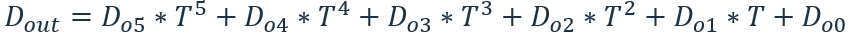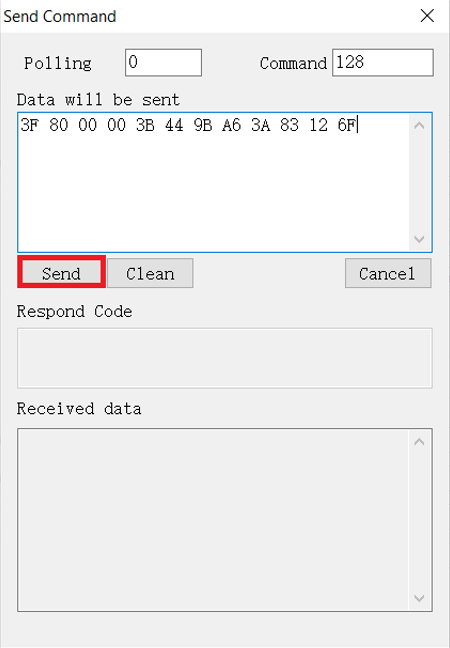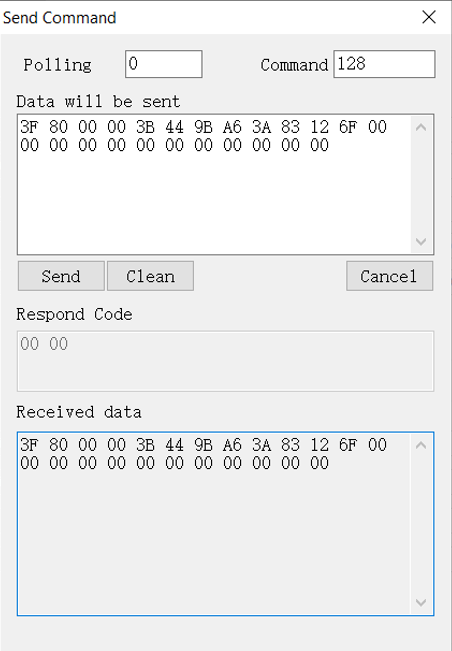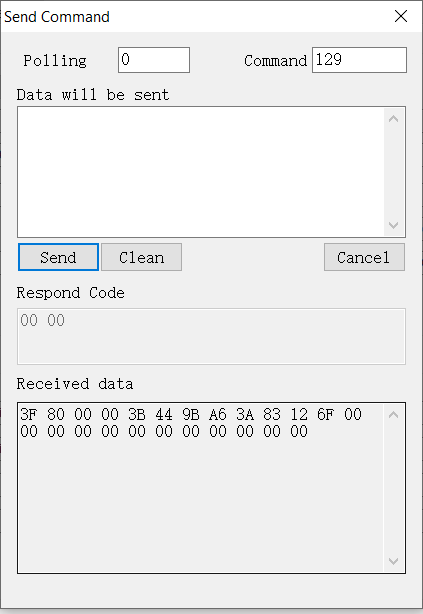This article covers the usage of the HART-specific commands available for Rheonics sensors. Only SRV

### `1. Command 128-Write SRV Density`

This command is available in HART-7 (only), it accepts 6 Float values which are the Do0 to Do5(Coefficients from a temperature compensated density).

Request Data Bytes

Table 1

Bytes

Format

Data

0-3

Float

Do0

4-7

Float

Do1

8-11

Float

Do2

12-15

Float

Do3

16-19

Float

Do4

20-23

Float

Do5

Response data bytes

 Same as request data bytes.

Command specific response codes

 Code Class Description 0 Success Command accepted, does not guarantee that data will be accepted by the sensor 5 Error Command frames too short 7 Error The device is not an SRV and thus does not accept density parameters 64 Error Command not implemented (it's only available in HART-7)

Example:

The Density SRV output can be described as a polynomial of the form:(1)

This model can describe constant density or a temperature-dependent density.

a. Constant density input

To describe a constant density, the coefficient Do0 should be set equal to the constant density value, and all the other coefficients should be set to zero.

b. Temperature-dependent density input

To describe a temperature-dependent density, assign values to the coefficients, that reflects the density behavior of your fluid with temperature. If you have a fluid reference table (for example Cannon fluids, Nippon grease), you can fit the density to a polynomial model to get the coefficients.

All the tests below will be completed with HARTmpt software

`Command 128 - Write SRV Density`

SRV HART devices can edit these coefficients by using the HART command 128, this can be achieved by using the coefficient in hex format.

### 1. Define the coefficient values based on a polynomial model, for example

 Do0= 1 Do1=0.003 Do2=0.001

### 2. Convert coefficients to Hex based on the request data bytes from the table 1 from section 1, all unused values must be covered too, otherwise, the command will return an error command

 Do0= 0x3f800000 Do1=0x3b449ba6 Do2=0x3a83126f

### 4. Input data bytes with software like HARTmpt and click send commandFigure 1. Command 128, write density.

### 5. Command 128 response, the response is as expected based on Table 1Figure 2. Command 128 response from HARTmpt.

### `2. Command 129-Read SRV density`

This command is available in HART-7 (only), it accepts 6 Float values which are the Do0 to Do5(Coefficients from a temperature compensated density)

Request Data Bytes

 There are no request data bytes

Response data bytes

 See response from the status from each coefficient from density

Command specific response codes

 There are not command specific responses

Command 129 will return the current values from the density model that are loaded into the SRVFigure 3. Command 129 response.